The Theory \ Details \ portfolio analytics \ historical portfolio

# Portfolio Analytics

## Historical portfolio

Let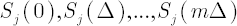denote discounted prices of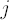-th portfolio component at respective times, where.
Symbols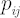and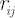denote respectively
simple return and logarithmic return in-th portfolio component over the period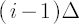to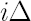.

Consider a portfolio with constant weights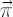. It means that at the end of each period such portfolio is rebalanced to state.

### Portfolio Dynamics

Symbols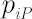anddenote respectively simple return and logarithmic return in discounted portfolio wealth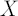over the periodto. Then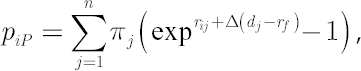where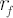denotes the
risk-free rate;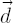denotes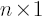vector of
dividend yields.
Accordingly,.
Thus, the discounted portfolio wealth at timeis equal to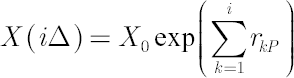, wheredenotes initial wealth.

### Historical Portfolio Excess Growth Rate

Historical portfolio excess growth rate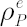is equal to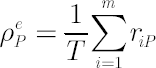.

Note. When using SmartFolio it might appear that for the same portfoliovalue of the
historical portfolio excess growth rate differ substantially from the expected excess growth rate, calculated
under the
analytical model assumptions. There are three reasons that explain such deviation:
• Parameters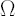and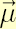used in analytical portfolio don’t correspond to the historical data. It happens when portfolio components have different lengths of historical data; analyzed time period in parameters
estimation settings doesn’t coincide with historical one;
sample estimates are modified by some of more advanced estimation methods.
• Distribution of log returns for some assets in portfolio significantly deviates from normality. This is often the case when hedge funds or derivatives are included in the portfolio.
• Rebalancing period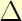is sufficiently long to violate the approximation of an analytical portfolio, which is rebalanced continually, with a historical simulation, where rebalancing takes place at the end of everyperiod.

### Historical Portfolio Volatility

Historical portfolio volatilityis defined as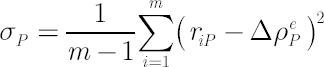### Historical Portfolio Excess Mu

Historical portfolio excess Muis equal to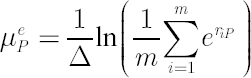.

### Contribution to Portfolio Risk

For calculation of vector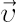of portfolio components contributions to risk SmartFolio utilizes the following approximate formula: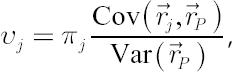where,. It is easy to see that for sufficiently smallquantityis close to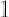.